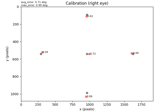# mne.preprocessing.eyetracking.Calibration#

class mne.preprocessing.eyetracking.Calibration(*, onset, model, eye, avg_error, max_error, positions, offsets, gaze, screen_size=None, screen_distance=None, screen_resolution=None)[source]#

Eye-tracking calibration info.

This data structure behaves like a dictionary. It contains information regarding a calibration that was conducted during an eye-tracking recording.

Note

When possible, a Calibration instance should be created with a helper function, such as `read_eyelink_calibration()`.

Parameters
onset`float`

The onset of the calibration in seconds. If the calibration was performed before the recording started, the the onset can be negative.

model`str`

A string, which is the model of the eye-tracking calibration that was applied. For example `'H3'` for a horizontal only 3-point calibration, or `'HV3'` for a horizontal and vertical 3-point calibration.

eye`str`

The eye that was calibrated. For example, `'left'`, or `'right'`.

avg_error`float`

The average error in degrees between the calibration positions and the actual gaze position.

max_error`float`

The maximum error in degrees that occurred between the calibration positions and the actual gaze position.

positionsarray_like of `float`, shape `(n_calibration_points, 2)`

The x and y coordinates of the calibration points.

offsetsarray_like of `float`, shape `(n_calibration_points,)`

The error in degrees between the calibration position and the actual gaze position for each calibration point.

gazearray_like of `float`, shape `(n_calibration_points, 2)`

The x and y coordinates of the actual gaze position for each calibration point.

screen_sizearray_like of shape `(2,)`

The width and height (in meters) of the screen that the eyetracking data was collected with. For example `(.531, .298)` for a monitor with a display area of 531 x 298 mm.

screen_distance`float`

The distance (in meters) from the participant’s eyes to the screen.

screen_resolutionarray_like of shape `(2,)`

The resolution (in pixels) of the screen that the eyetracking data was collected with. For example, `(1920, 1080)` for a 1920x1080 resolution display.

Methods

 `__contains__`(key, /) True if the dictionary has the specified key, else False. `__getitem__` x.__getitem__(y) <==> x[y] Implement iter(self). Return len(self). Copy the instance. `fromkeys`(iterable[, value]) Create a new dictionary with keys from iterable and values set to value. `get`(key[, default]) Return the value for key if key is in the dictionary, else default. `plot`([show_offsets, axes, show]) Visualize calibration. `pop`(key[, default]) If the key is not found, return the default if given; otherwise, raise a KeyError. Remove and return a (key, value) pair as a 2-tuple. `setdefault`(key[, default]) Insert key with a value of default if key is not in the dictionary. `update`([E, ]**F) If E is present and has a .keys() method, then does: for k in E: D[k] = E[k] If E is present and lacks a .keys() method, then does: for k, v in E: D[k] = v In either case, this is followed by: for k in F: D[k] = F[k]
__contains__(key, /)#

True if the dictionary has the specified key, else False.

__getitem__()#

x.__getitem__(y) <==> x[y]

__iter__(/)#

Implement iter(self).

__len__(/)#

Return len(self).

clear() None.  Remove all items from D.#
copy()[source]#

Copy the instance.

Returns
calinstance of `Calibration`

The copied Calibration.

fromkeys(iterable, value=None, /)#

Create a new dictionary with keys from iterable and values set to value.

get(key, default=None, /)#

Return the value for key if key is in the dictionary, else default.

items() a set-like object providing a view on D's items#
keys() a set-like object providing a view on D's keys#
plot(show_offsets=True, axes=None, show=True)[source]#

Visualize calibration.

Parameters
show_offsets`bool`

Whether to display the offset (in visual degrees) of each calibration point or not. Defaults to `True`.

axesinstance of `matplotlib.axes.Axes` | `None`

Axes to draw the calibration positions to. If `None` (default), a new axes will be created.

show`bool`

Whether to show the figure or not. Defaults to `True`.

Returns
figinstance of `matplotlib.figure.Figure`

The resulting figure object for the calibration plot.

Examples using `plot`:Working with eye tracker data in MNE-Python

Working with eye tracker data in MNE-Python
pop(key, default=<unrepresentable>, /)#

If the key is not found, return the default if given; otherwise, raise a KeyError.

popitem(/)#

Remove and return a (key, value) pair as a 2-tuple.

Pairs are returned in LIFO (last-in, first-out) order. Raises KeyError if the dict is empty.

setdefault(key, default=None, /)#

Insert key with a value of default if key is not in the dictionary.

Return the value for key if key is in the dictionary, else default.

update([E, ]**F) None.  Update D from dict/iterable E and F.#

If E is present and has a .keys() method, then does: for k in E: D[k] = E[k] If E is present and lacks a .keys() method, then does: for k, v in E: D[k] = v In either case, this is followed by: for k in F: D[k] = F[k]

values() an object providing a view on D's values#

## Examples using `mne.preprocessing.eyetracking.Calibration`#Working with eye tracker data in MNE-Python

Working with eye tracker data in MNE-Python